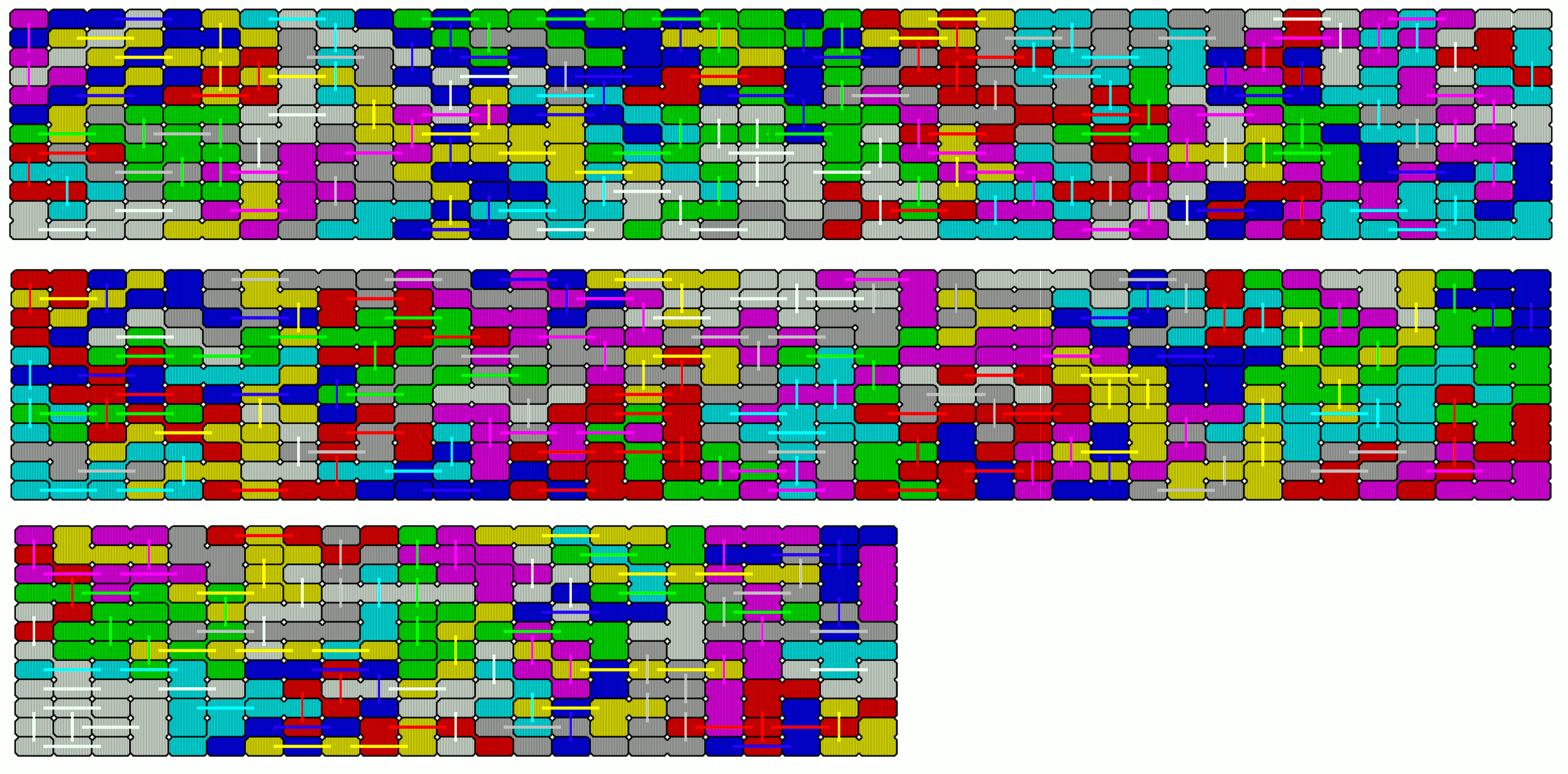Three Connected Rectangles

There are 19 two-sided pieces with a total area of 57 rectangles. For special side ratios of the rectangles constructions out of the rectangle grid may be possible, but these constructions are only valid for the given special side ratio.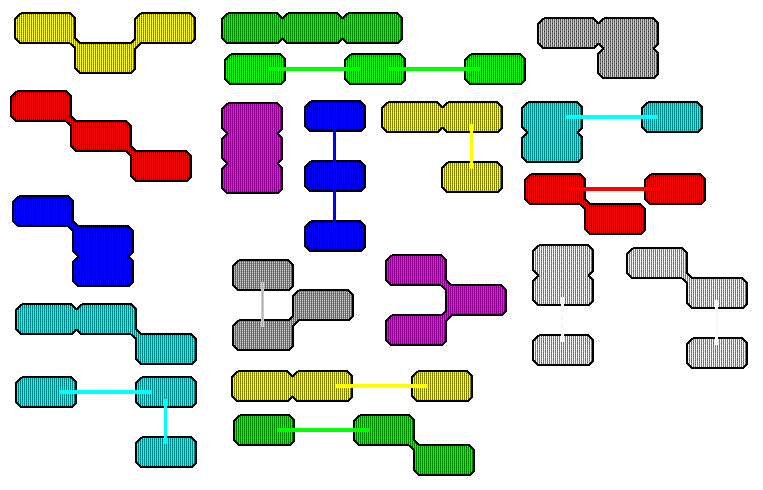A 19x3 rectangle can't be made with these pieces, because one piece (a violet one) has a height of 5 rectangles and doesn't fit. A piece with width 5 (a green one) prevents the 3x19 rectangle.

But there are various constructions with two axes of symmetry.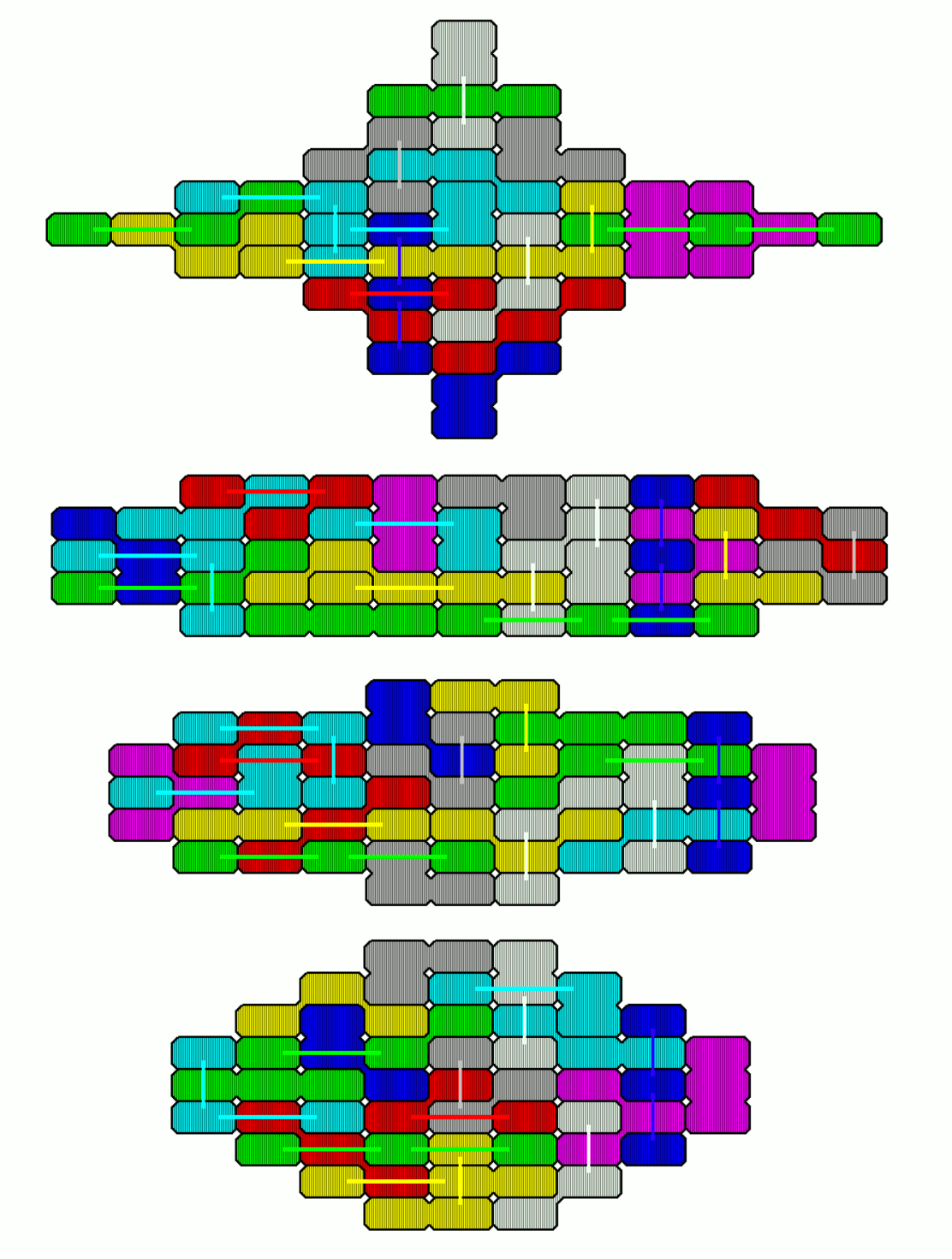If we add six grid elements we have a total area of 63 and can construct 7x9 rectangles with holes of six rectangles connected at their sides like hexominoes.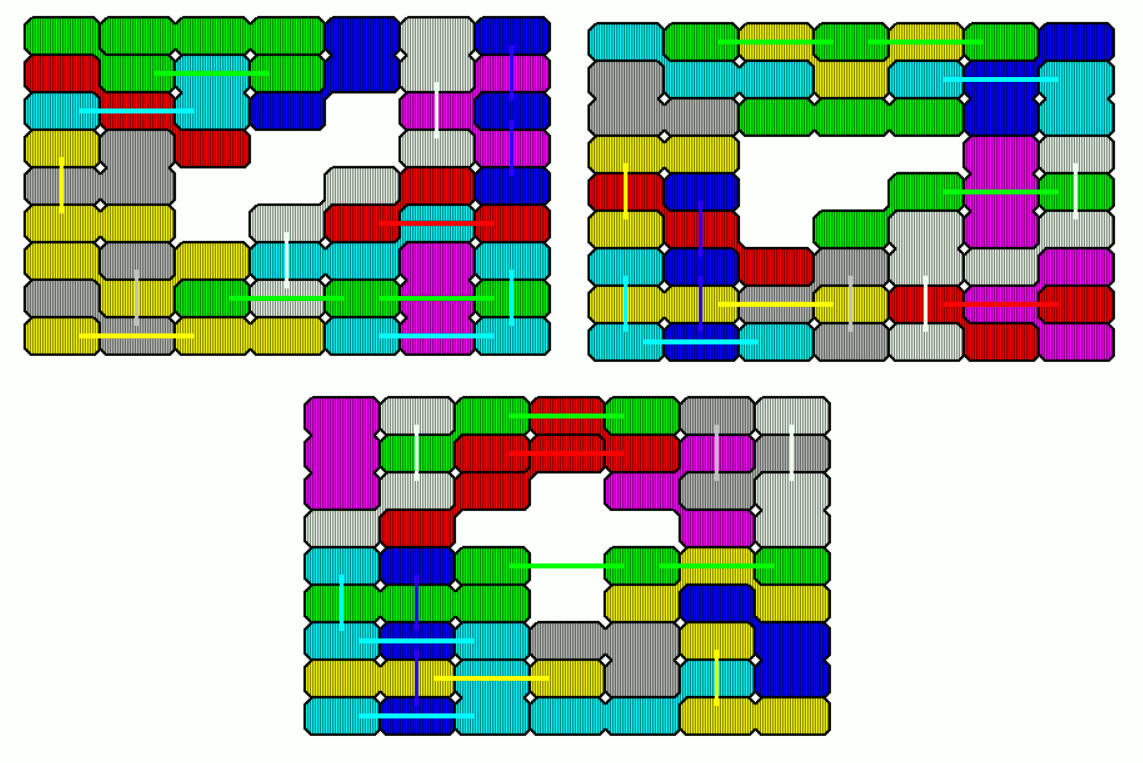If the grid cells have a side ratio of 1:2 we can make a rectangle using grid change.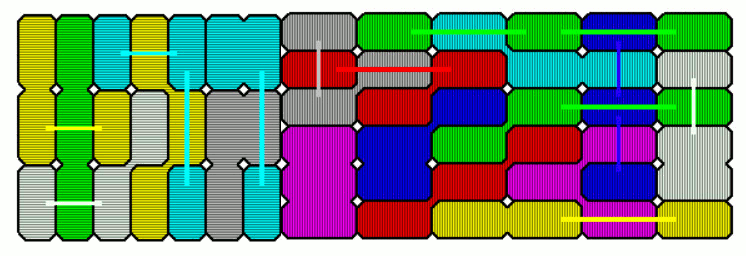The set of one-sided pieces with three connected rectangles has 30 elements and a total area of 90 rectangles. The obj-files of the printed pieces are here, but solving problems by hand seems to be more difficult for this set.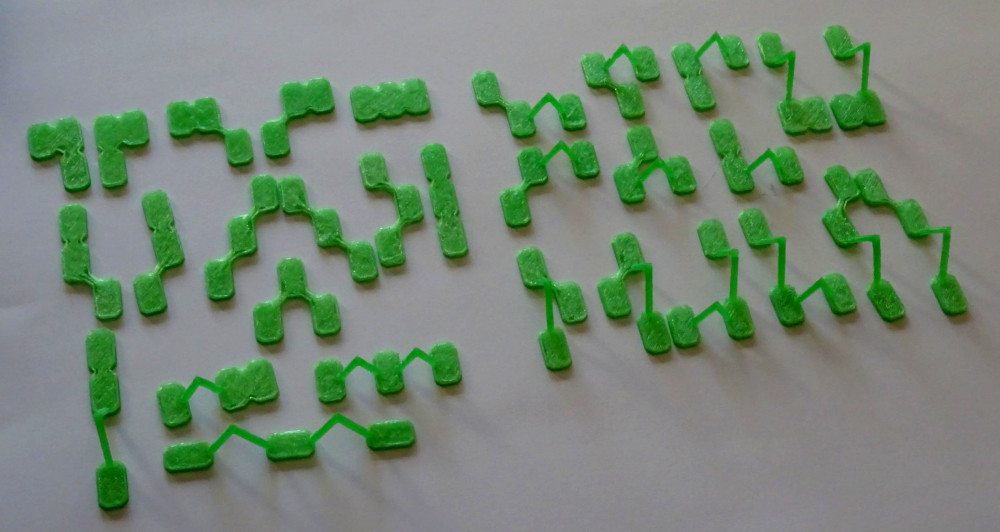Rectangles of size 5x18, 6x15 and 9x10 are possible.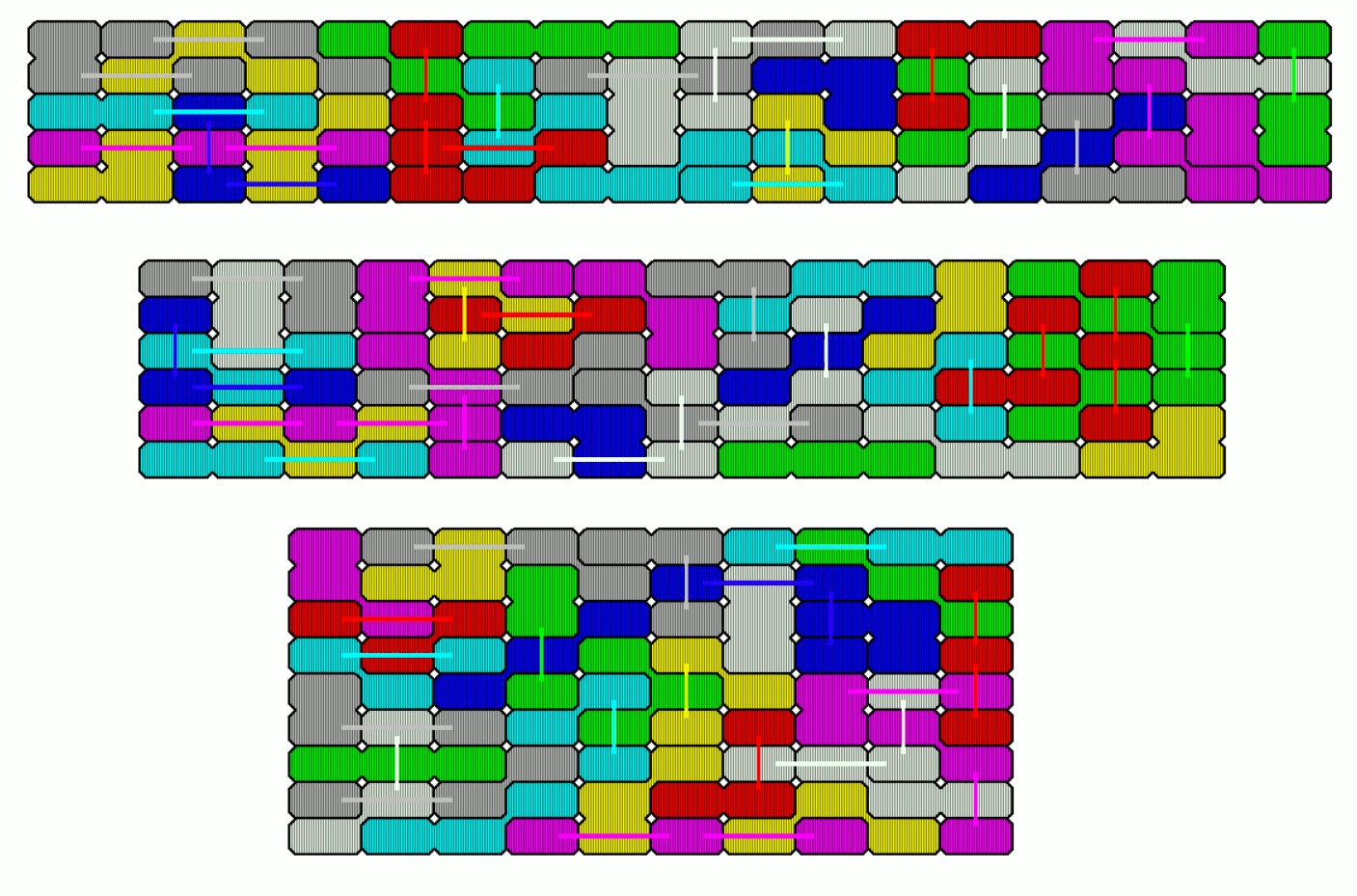Furthermore you can get rectangles with rectangular holes.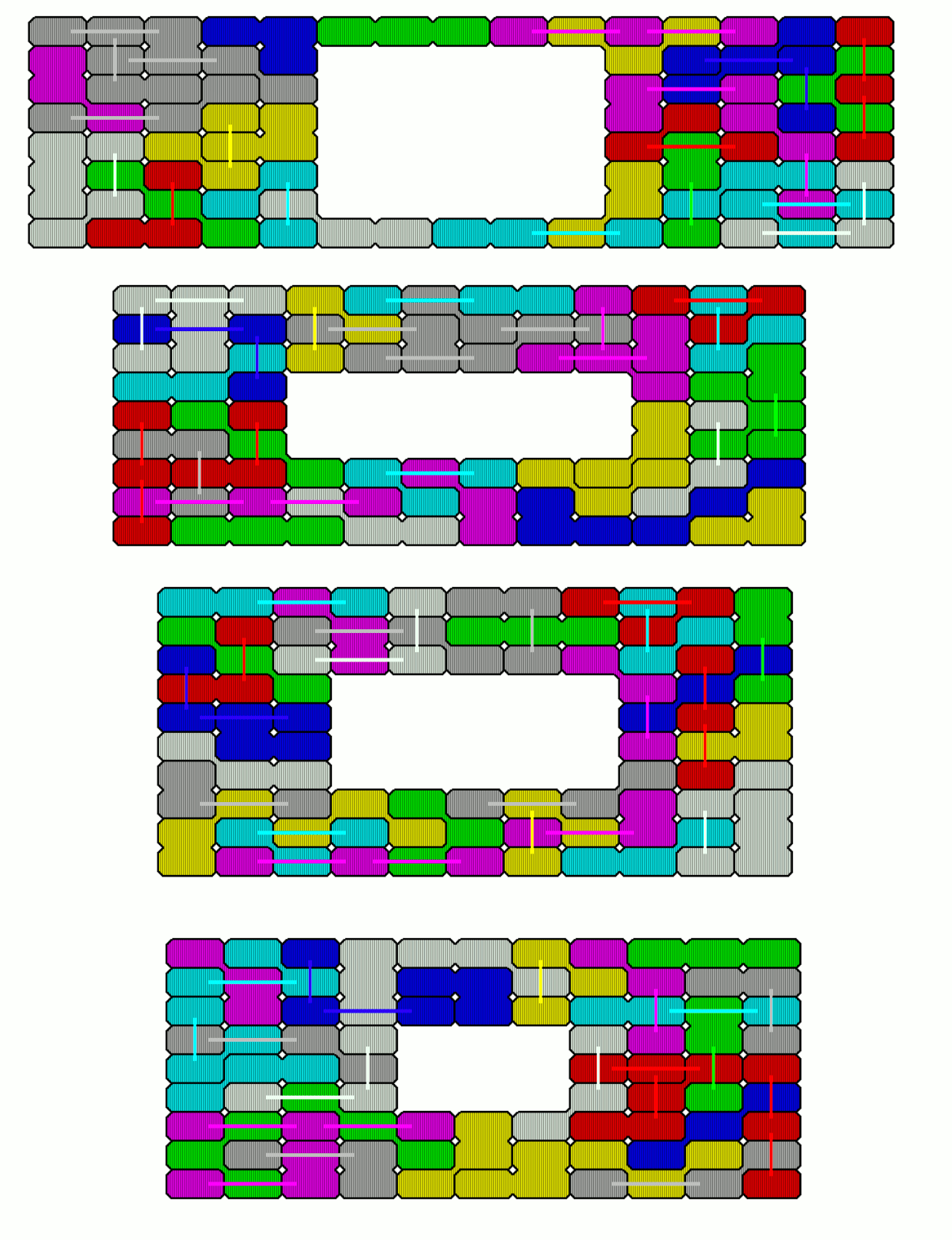I looked for figures with two axes of symmetry.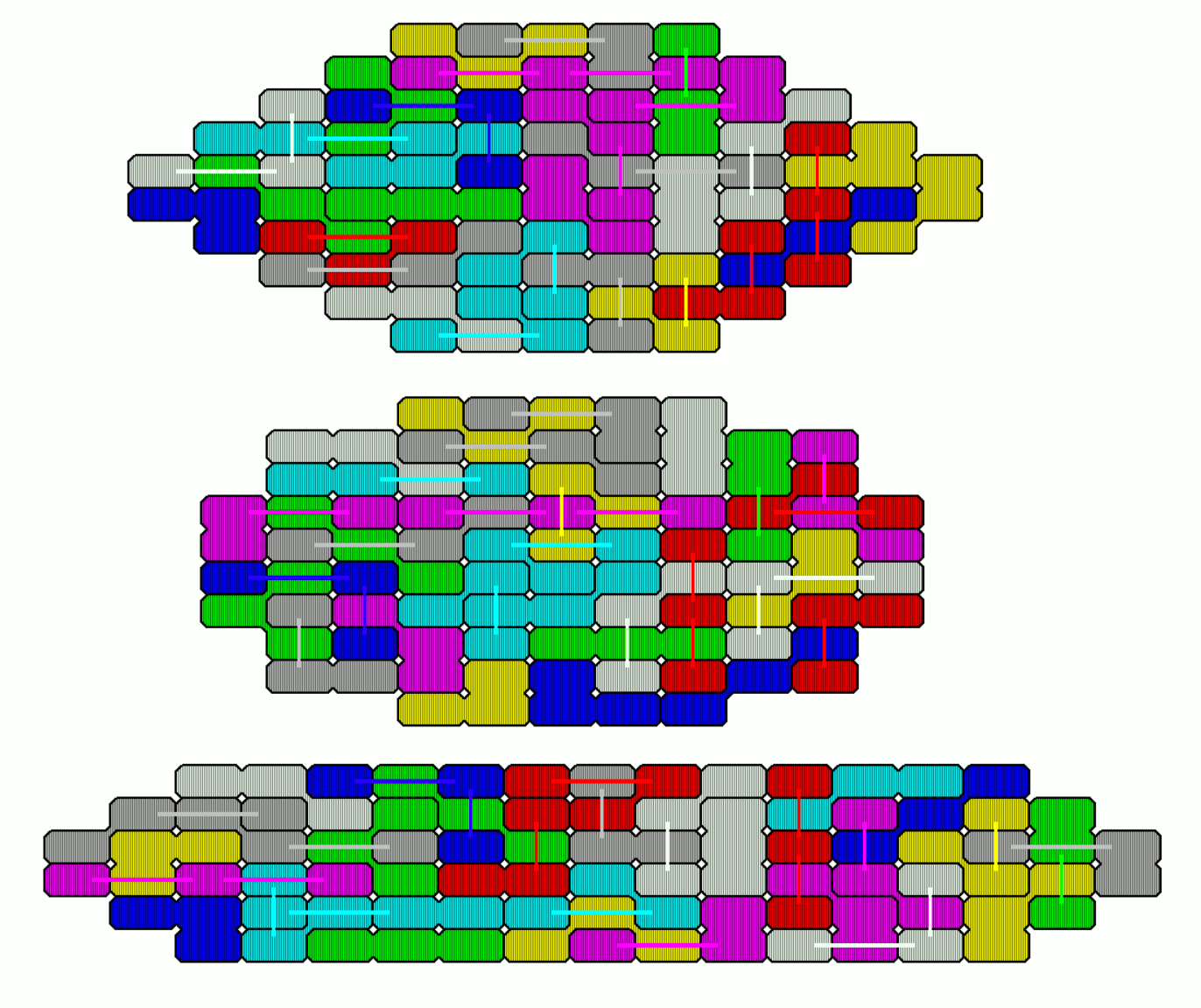Pentarectangles are rectangles connected at their sides. Holes shaped like pentarectangles can be placed in some rectangles.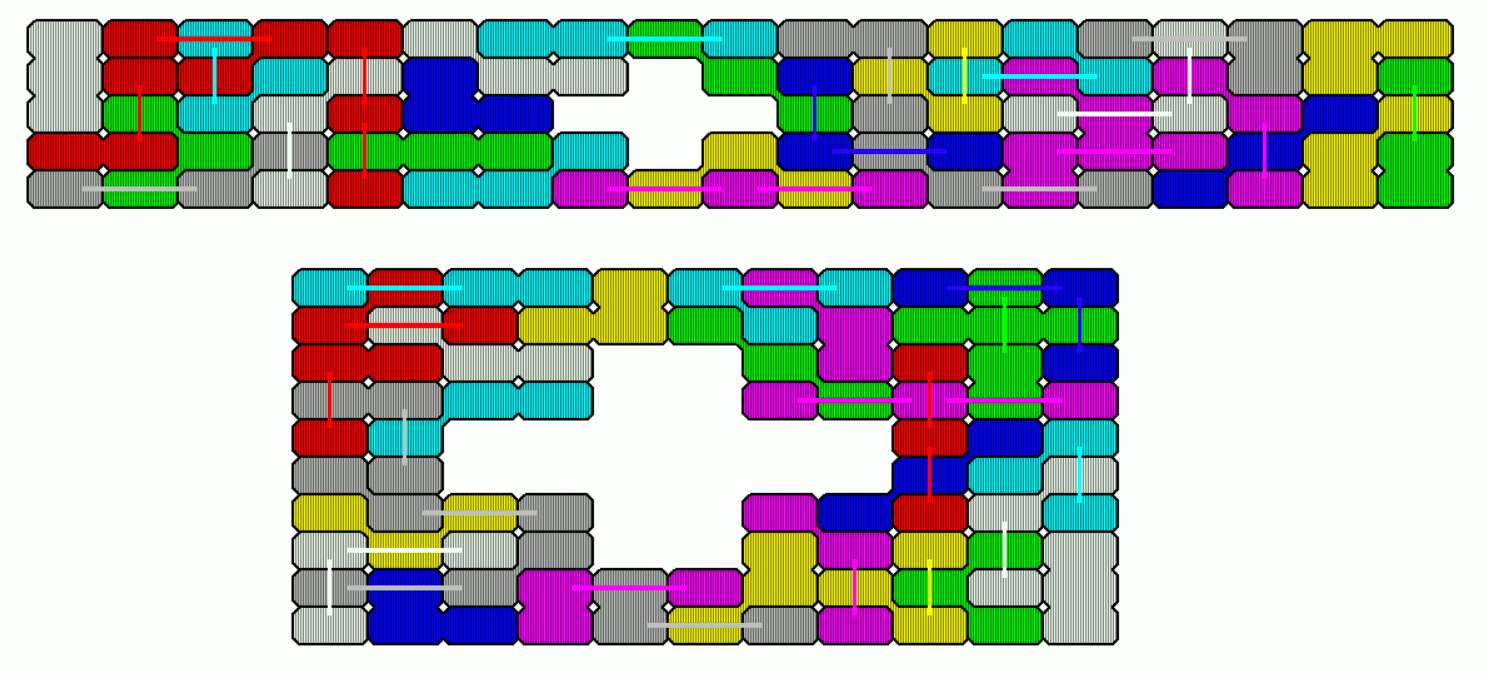If the side ratio of the rectangles is 1:2, models of the pentominoes can be constructed.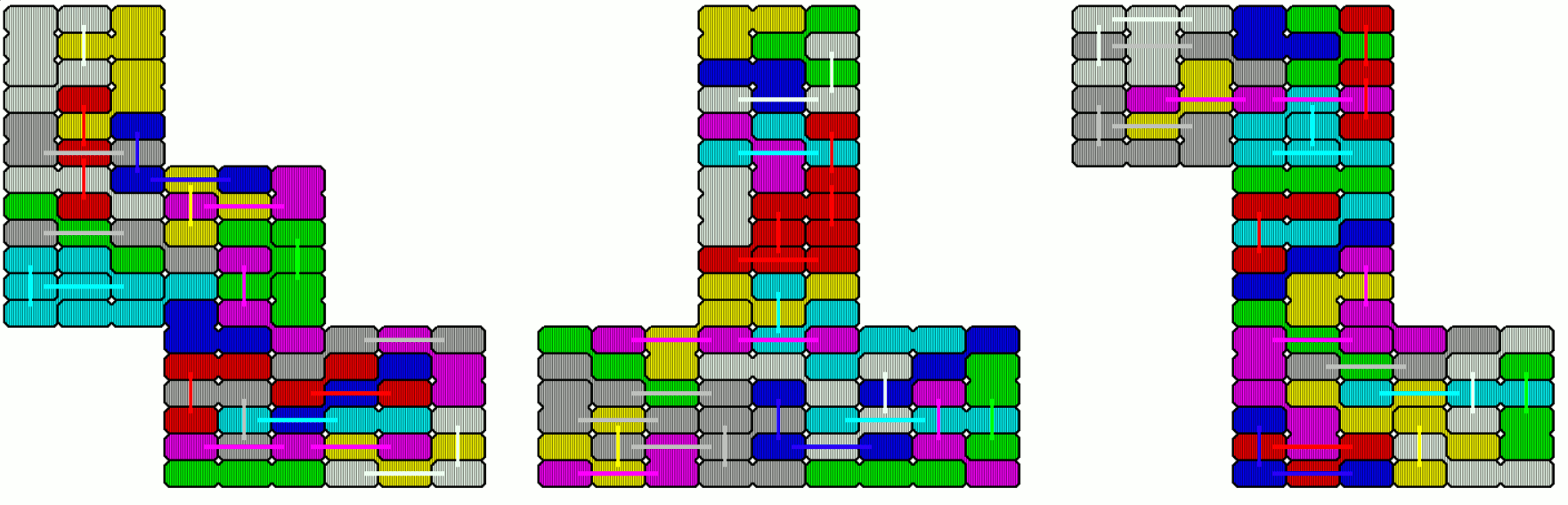With the given printed pieces only models of pentarectangles are possible.Four Connected Rectangles

There are 173 two-sided pieces with a total area of 692 rectangles, but we have a parity problem. Under checkerboard coloring there are 62 pieces with an odd number of black and white rectangles. That's ok. But let's alternately color the rows of a rectangle. This way we get an odd number of pieces with odd numbers of black and white parts. Since we can't turn the pieces by 90 degrees we always have an odd number of black and white rectangles, but a large rectangle with 692 small rectangles has an even number of small rectangles. Therefore a construction is impossible. I discarded a L-tetrarectangle and withh the remaining 172 pieces I got a 8x86 rectangle by solving two 8x43 parts.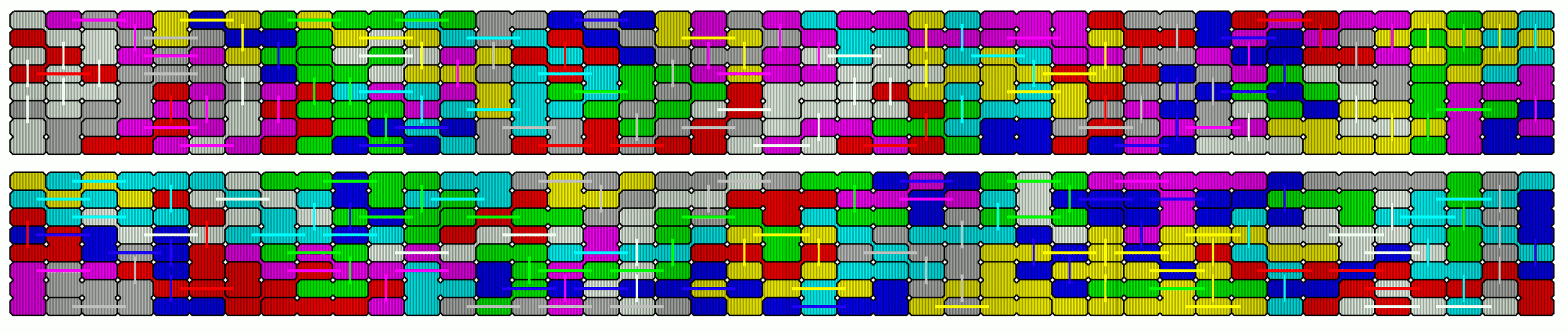The one-sided 319 tetrarectangles with an area of 1240 have the same parity problem as the two-sided set. Without a L-tetrarectangle I solved two 12x40 and one 12x23 rectangles to get a large rectangle of size 12x103 = 1236.